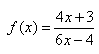# Test: Problems On Composition

## 10 Questions MCQ Test Mathematics (Maths) Class 12 | Test: Problems On Composition

Description
This mock test of Test: Problems On Composition for JEE helps you for every JEE entrance exam. This contains 10 Multiple Choice Questions for JEE Test: Problems On Composition (mcq) to study with solutions a complete question bank. The solved questions answers in this Test: Problems On Composition quiz give you a good mix of easy questions and tough questions. JEE students definitely take this Test: Problems On Composition exercise for a better result in the exam. You can find other Test: Problems On Composition extra questions, long questions & short questions for JEE on EduRev as well by searching above.
QUESTION: 1

### If f(x) = ax + b and g(x) = cx + d, then f[g(x)] – g[f(x)] is equivalent to​

Solution:

We have f(x) = ax+b, g(x) = cx+d
Therefore, f{g(x)} = g{f(x)}
⇔ f(cx+d) = g(ax+b)
⇔ a(cx+d)+b = c(ax+b)+d
⇔ f(d) - g(b)

QUESTION: 2

### If, then inverse of f is:

Solution:

let y = (4x + 3)/(6x - 4)
⇒ y * (6x - 4) = 4x + 3
⇒ 6xy - 4y = 4x + 3
⇒ 6xy - 4y - 4x = 3
⇒ 6xy - 4x = 3 + 4y
⇒ x(6y - 4) = 4y + 3
⇒ x = (4y + 3)/(6y - 4)
So, f-1 (x) = (4x + 3)/(6x - 4)

QUESTION: 3

### If f(x) = (3 – x2)1/2, then fof is:​

Solution:
QUESTION: 4

If f: R → R and g: R → R be defined as f(x) = x + 1 and g(x) = x – 1. Then for all x ∈ R​

Solution:

Given f:R→R and g: R→R
=> fog : R→R and gof :  R→R
We know that I(R) : R→R
So the domains of gof, fog I(R) are the same.
fog(x) = f(g(x)) = f(x-1) = x-1+1 = I(R)x…..(1)
gof(x) = g(f(x)) = g(x-1) = x-1+1 = I(R)x……(2)
From (1) and (2), we get
fog(x) = gof(x) = I(R) for all x belongs to R
Hence fog = gof

QUESTION: 5

If f and g two functions such that they are one-one then g o f is

Solution:

f:A→B and g:B→C are both one-to-one functions.
Suppose a1,a2∈A such that (gof)(a1)=(gof)(a2)
⇒ g(f(a1)) = g(f(a2)) (definition of composition)
Since gg is one-to-one, therefore,
f(a1) = f(a2)
And since ff is one-to-one, therefore,
a1 = a2
Thus, we have shown that if (gof)(a1)=(gof)(a2 then a1=a2
Hence, gof is one-to-one function.

QUESTION: 6

Let A = {a,b,c} and B = {1,2,3} and f: A→B is defined by f={(a,2), (b,1), (c,3)}. Find f-1

Solution:
QUESTION: 7

If f: A→B and g:B→C are onto, then gof:A→C is:​

Solution:

since g : B → C is onto
Suppose z implies C, there exist a pre image in B
Let the pre image be y
Hence y implies B such that g(y) = z
Similarly  f : A → B is onto
Suppose y implies B, there exist a pre image in A
Let the pre image be x
Hence y implies A such that f(x) = y
Now, gof : A → C gof = g(f(x))
= g(y)
= z
So, for every x in A, there is an image z in C
Thus, gof is onto.

QUESTION: 8

A function in which second element of order pair are distinct is called

Solution:
QUESTION: 9

If f be a mapping defined by f(x) = x2 + 5, then  f-1 (x) is:

Solution:

f(x) = x2 + 5 = y(let)
⇒ y = x2+5
⇒ x2 = y-5
⇒ x = (y-5)1/2
⇒ f-1(x) = (x-5)1/2

QUESTION: 10

Let A = {2 , 3 , 6}. Which of the following relations on A are reflexive?

Solution:

R1 is a reflexive on A , because (a,a) ∈ R1  for each  a ∈ A